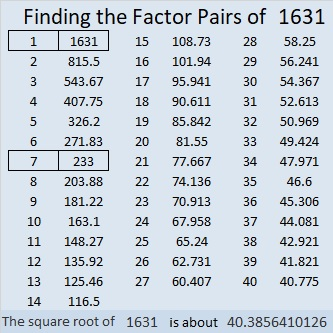# 1631 The Importance of Practice

### Today’s Puzzle:

I did not have the privilege of learning a musical instrument when I was growing up, but I did make sure my children had that opportunity. One of the topics discussed in this next episode of Bill Davidson’s Podcast is the importance that practice plays in both music and mathematics. I thought it was quite good.

I think practicing is best when it is enjoyable. If you solve this musical note puzzle, it will hopefully be an enjoyable way for you to practice a few multiplication and division facts. Just use logic to write the numbers from 1 to 10 in both the first column and the top row so that those numbers and the given clues will function like a multiplication table.### Factors of 1631:

• 1631 is a composite number.
• Prime factorization: 1631 = 7 × 233.
• 1631 has no exponents greater than 1 in its prime factorization, so √1631 cannot be simplified.
• The exponents in the prime factorization are 1 and 1. Adding one to each exponent and multiplying we get (1 + 1)(1 + 1) = 2 × 2 = 4. Therefore 1631 has exactly 4 factors.
• The factors of 1631 are outlined with their factor pair partners in the graphic below.### More about the Number 1631:

1631 is the hypotenuse of a Pythagorean triple:
735-1456-1631, which is 7 times (105-208-233).

1631 is the difference of two squares in two different ways:
816² – 815² = 1631, and
120² – 113² = 1631.

I found those number facts just from looking at the factors of 1631.